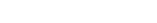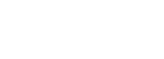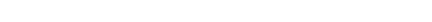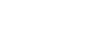# GMAT Math: Can you divide by a variable?In this edition of GMAT Algebra, we’re going over the answer to: “Can you divide by a variable?” Keep reading to get our explanation and some examples of this concept in action.

Question #1: In the equation,can you divide both sides by x?

Question #2: In the equation (x – 3)(x + 5) = (2x + 1)(x + 5), can you divide both sides by (x + 5)?

Question #3:Question #4:## Dividing by a variable or by an algebraic expression

You see, it’s mathematically illegal to divide by zero, and if you don’t know the value of the variable, then you could be breaking the law without knowing it.  Ask any judge — not knowing that you’re breaking the law generally is not an excuse that holds very well up in court.  In much the same way, not knowing whether you are dividing by zero, because you are dividing by an unknown, is just as bad as dividing by zero directly.

What do you do instead?  Well, there are two alternatives.  One method is: instead of dividing by the variable, factor it out.  For example, with Question #1:If the produce of two or three or more factors equals zero, this means one of the factors must equal zero.  Here, either x = 0 or (x + 3) = 0, which leads to solutions of x = 0 and x = –3.

The second method is two break the problem into two cases, one in which the variable or expression does equal zero, and one in which it doesn’t.  Treat the two cases separate.  For example, in Question #2:

Case I: let’s consider the case in which (x + 5) = 0.  Well, if this equaled zero, the equation would be true, so this is a solution.  One solution is x = –5.

Case II: let’s consider the case in which (x + 5) ≠ 0, that is, the case in which x ≠ –5.  Well, now we are guaranteed that  (x + 5) ≠ 0 is not equal to zero, so dividing both sides by this expression is now perfectly legal, and this leads to the simple equation x – 3 = 2x + 1, which has a solution of x = –4.  Thus, the overall solutions to this problem are x = –5 and x = –4.

## Canceling a variable or expression

Similarly, the blanket answer to the cancelling question is also, NO!, for the same reason.  If there is any possibility that your variable or expression equals zero, then cancelling would be a 100% illegal activity.

For Question #3 — for all values of x other than x = 0, for the entire continuous infinity of numbers on the number line excluding that solitary value, yes, the fraction 2x/5x would equal 2/5.  BUT, when x = 0, that statement is no longer true — it is not even false — it is profoundly meaningless.  It would be like asking whether the number 163 has a flavor — even posing the question implies a profound misconstruing of essential nature of what a number is.  For this one, we would have to say — whatever the question is asking, whatever the question is doing, we have to recognize that x = 0 is not at all a possible value; having eliminating that value, we can proceed with whatever the rest of the problem may be.

Question #4 is a particularly interesting one.  First of all, as with the previous example, we run into major difficulties when the factor-to-be-cancelled equals zero.  As with the other questions, we can’t just do a blanket cancelling with impunity.   As with the previous two questions, we have to consider cases.  If (x + 2) = 0, then the expression on the left becomes 0/0, profoundly meaningless, and any statement setting this equal to anything else would be sheer nonsense.  If (x + 2) = 0, then nothing equals anything else in this problem, so x = –2 is definitely not a legitimate answer.

Now, what happens in the case in which (x + 2) ≠ 0?  Well, in this case, this factor does not equal zero, so it can be cancelled, which leads to:Now, we have the same expression on both sides of the equation.  This means, these two sides would be equal for all values of x, as long as the expression is defined.  This means the whole continuous infinity of the number line is legal, barring a couple isolated exceptions.  One is x = –4, which makes the denominator equal zero — something divided by zero cannot equal anything, because something divided by zero has already departed from the realm in which any mathematically meaningful statement is possible.  And, of course, as we discovered above, x = –2 cannot be a solution either.  Therefore, the solution consists of all real numbers, the entire continuous infinity of the real number line, except for the values x = –4 and x = –2.

## Summary

Don’t divide by variables or by algebraic expressions.  Don’t cancel by variables or by algebraic expressions.  Always consider whether the factor by which you would want to divide could equal zero, and either factor it out or consider the process in separate cases.

## Author

•Mike served as a GMAT Expert at Magoosh, helping create hundreds of lesson videos and practice questions to help guide GMAT students to success. He was also featured as "member of the month" for over two years at GMAT Club. Mike holds an A.B. in Physics (graduating magna cum laude) and an M.T.S. in Religions of the World, both from Harvard. Beyond standardized testing, Mike has over 20 years of both private and public high school teaching experience specializing in math and physics. In his free time, Mike likes smashing foosballs into orbit, and despite having no obvious cranial deficiency, he insists on rooting for the NY Mets. Learn more about the GMAT through Mike's Youtube video explanations and resources like What is a Good GMAT Score? and the GMAT Diagnostic Test.